# PH_meter_SKU__SEN0161_-DFRobot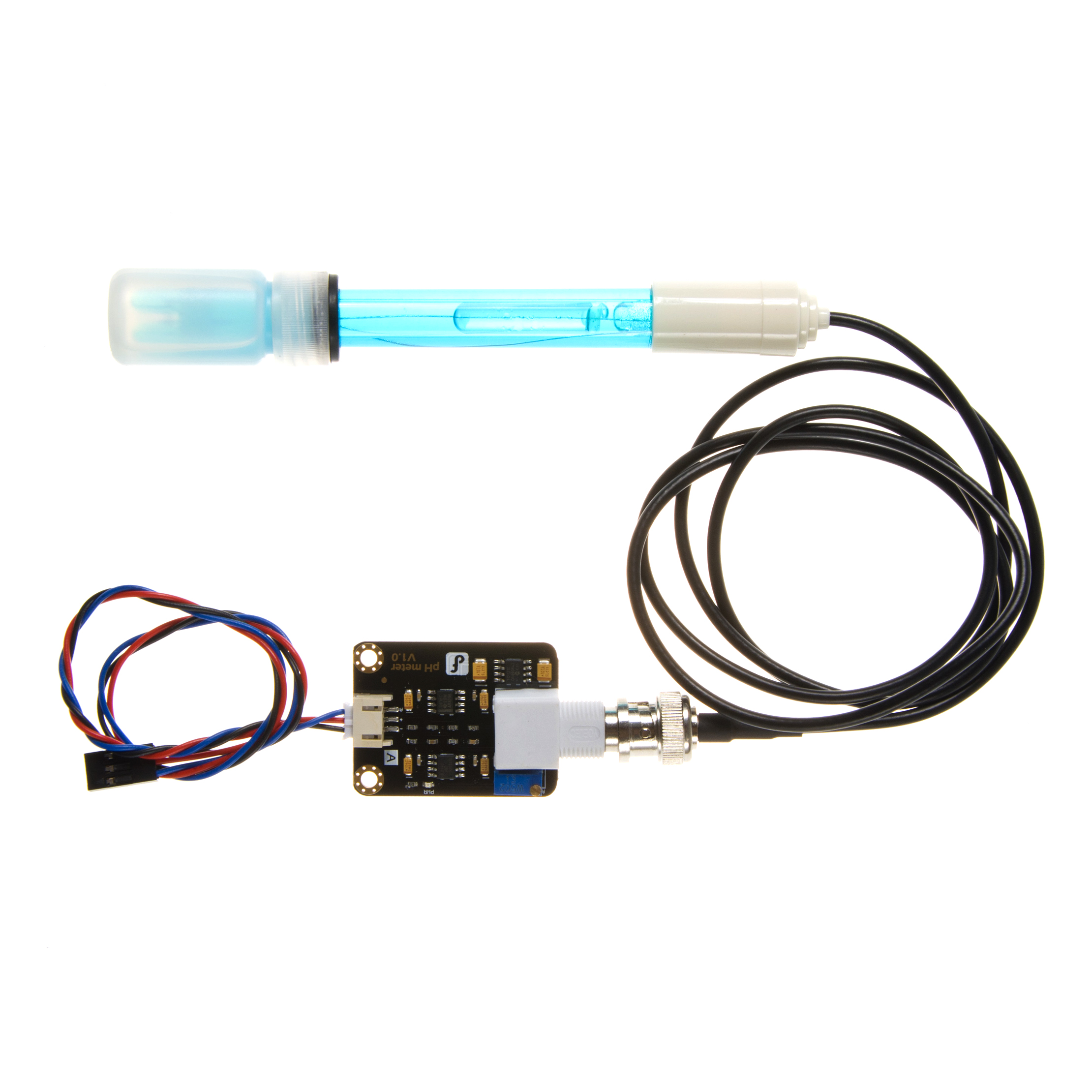## Introduction

Need to measure water quality and other parameters but haven't got any low cost pH meter? Find it difficult to use with Arduino? Here comes an analog pH meter, specially designed for Arduino controllers and has built-in simple, convenient and practical connection and features. It has an LED which works as the Power Indicator, a BNC connector and PH2.0 sensor interface. To use it, just connect the pH sensor with BNC connector, and plug the PH2.0 interface into the analog input port of any Arduino controller. If pre-programmed, you will get the pH value easily. Comes in compact plastic box with foams for better mobile storage. Attention:In order to ensure the accuracy of the pH probe, you need to use the standard solution to calibrate it regularly.Generally, the period is about half a year. If you meaure the dirty aqueous solution, you need to increase the frequency of calibration.

## Applications

• Water quality testing
• Aquaculture

## Specification

• Module Power : 5.00V
• Module Size : 43mm×32mm
• Measuring Range:0-14PH
• Measuring Temperature :0-60 ℃
• Accuracy : ± 0.1pH (25 ℃)
• Response Time : ≤ 1min
• pH Sensor with BNC Connector
• PH2.0 Interface ( 3 foot patch )
• Power Indicator LED
• Cable Length from sensor to BNC connector:660mm

## Board Overview

### pH Electrode Size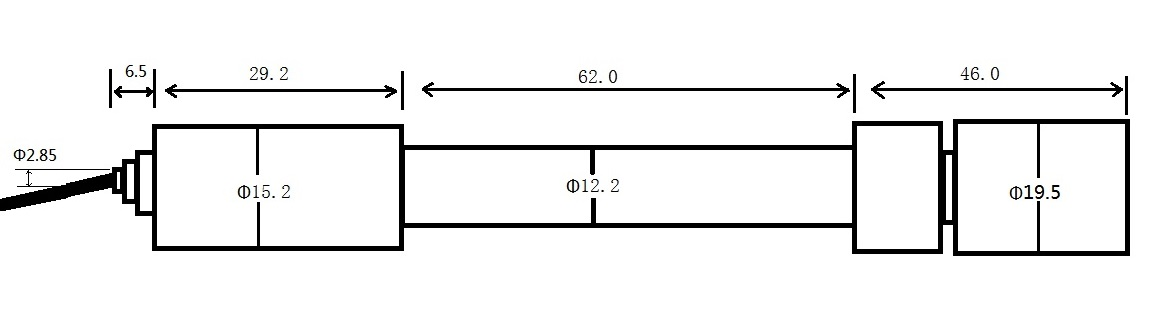### pH Electrode Characteristics

The output of pH electrode is Millivolts,and the pH value of the relationship is shown as follows (25 ℃):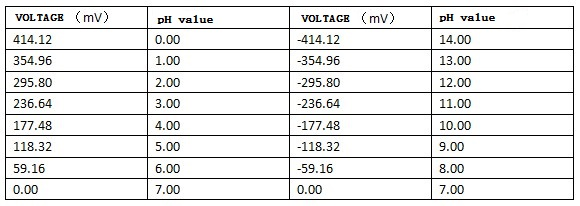## Tutorial

### Connecting Diagram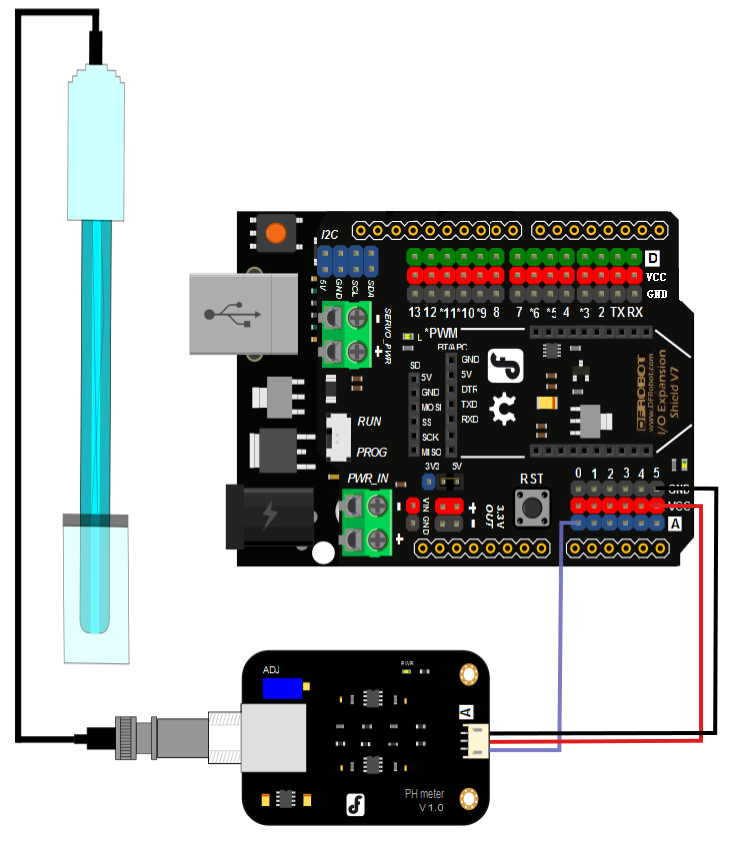### Step to Use the pH Meter

Cautions:

• Please use an external switching power supply,and the voltage as close as possible to the +5.00V. More accurate the voltage, more higher the accuracy!

• Before the electrode in continuous use every time,you need to calibrate it by the standard solution,in order to obtain more accurate results.The best environment temperature is about 25 ℃,and the pH value is known and reliable,close to the measured value. If you measure the acidic sample, the pH value of the standard solution should be 4.00.If you measure the alkaline sample, the pH value of the standard solution should be 9.18.Subsection calibration, just in order to get a better accuracy.

• Before the pH electrode measured different solutions, we need to use water to wash it. We recommend using deionized water.

(1)Connect equipments according to the graphic,that is,the pH electrode is connected to the BNC connector on the pH meter board，and then use the connection lines,the pH meter board is connected to the ananlong port 0 of the Arduino controller. When the Arduino controller gets power,you will see the blue LED on board is on. (2)Upload the sample code to the Arduino controller. (3)Put the pH electrode into the standard solution whose pH value is 7.00，or directly shorted the input of the BNC connector.Open the serial monitor of the Arduino IDE,you can see the pH value printed on it,and the error does not exceed 0.3. Record the pH value printed,then compared with 7.00, and the difference should be changed into the "Offset" in the sample code. For example,the pH value printed is 6.88,so the difference is 0.12.You should change the "# define Offset 0.00" into "# define Offset 0.12" in your program. (4)Put the pH electrode into the pH standard solution whose value is 4.00.Then wait about one minute,adjust the gain potential device, let the value stabilise at around 4.00.At this time,the acidic calibration has been completed and you can measure the pH value of an acidic solution. Note:If you want to measure the pH value of other solution,you must wash the pH electrode first! (5) According to the linear characteristics of pH electrode itself, after the above calibration,you can directly measure the pH value of the alkaline solution, but if you want to get better accuracy, you can recalibrate it. Alkaline calibration use the standard solution whose pH value is 9.18.Also adjust the gain potential device, let the value stabilise at around 9.18. After this calibration, you can measure the pH value of the alkaline solution.

### Sample Code

Sample code for testing the PH meter and get the sensor feedback from the Arduino Serial Monitor.

``````/*
# This sample code is used to test the pH meter V1.0.
# Editor : YouYou
# Ver    : 1.0
# Product: analog pH meter
# SKU    : SEN0161
*/
#define SensorPin A0            //pH meter Analog output to Arduino Analog Input 0
#define Offset 0.00            //deviation compensate
#define LED 13
#define samplingInterval 20
#define printInterval 800
#define ArrayLenth  40    //times of collection
int pHArray[ArrayLenth];   //Store the average value of the sensor feedback
int pHArrayIndex=0;
void setup(void)
{
pinMode(LED,OUTPUT);
Serial.begin(9600);
Serial.println("pH meter experiment!");    //Test the serial monitor
}
void loop(void)
{
static unsigned long samplingTime = millis();
static unsigned long printTime = millis();
static float pHValue,voltage;
if(millis()-samplingTime > samplingInterval)
{
if(pHArrayIndex==ArrayLenth)pHArrayIndex=0;
voltage = avergearray(pHArray, ArrayLenth)*5.0/1024;
pHValue = 3.5*voltage+Offset;
samplingTime=millis();
}
if(millis() - printTime > printInterval)   //Every 800 milliseconds, print a numerical, convert the state of the LED indicator
{
Serial.print("Voltage:");
Serial.print(voltage,2);
Serial.print("    pH value: ");
Serial.println(pHValue,2);
printTime=millis();
}
}
double avergearray(int* arr, int number){
int i;
int max,min;
double avg;
long amount=0;
if(number<=0){
Serial.println("Error number for the array to avraging!/n");
return 0;
}
if(number<5){   //less than 5, calculated directly statistics
for(i=0;i<number;i++){
amount+=arr[i];
}
avg = amount/number;
return avg;
}else{
if(arr<arr){
min = arr;max=arr;
}
else{
min=arr;max=arr;
}
for(i=2;i<number;i++){
if(arr[i]<min){
amount+=min;        //arr<min
min=arr[i];
}else {
if(arr[i]>max){
amount+=max;    //arr>max
max=arr[i];
}else{
amount+=arr[i]; //min<=arr<=max
}
}//if
}//for
avg = (double)amount/(number-2);
}//if
return avg;
}``````

### Precautions

• The electrode used for the first or long set without re-use, the electrode bulb and the sand core, immersed in the 3NKCL solution activated eight hours.

• The electrode plug should be kept clean and dry.

• Electrode reference solution is the 3NKCL solution.

• Measurement should be avoided staggered pollution between solutions, so as not to affect the accuracy of measurement.

• Electrode blub or sand core is defiled which will make PTS decline, slow response. So, it should be based on the characteristics of the pollutant, adapted to the cleaning solution, the electrode performance recovery.

• The electrode should not be long-term immersed in acid chloride solution.

## FAQ

Q&A Some general Arduino Problems/FAQ/Tips
A For any questions, advice or cool ideas to share, please visit the DFRobot Forum.

## More Documents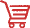Get Analog pH Sensor / Meter Kit For Arduino from DFRobot Store or DFRobot Distributor.